# Difference between revisions of "Estuarine circulation"

 Definition of Estuarine circulation: Residual flow pattern in an estuary induced by the density difference between seawater and river water. This is the common definition for Estuarine circulation, other definitions can be discussed in the article

Seawater has a higher density than river water, due to its high salinity. The density difference is of the order of 3%. In estuaries, where seawater and river water meet, seawater will tend to intrude beneath the river flow. However, mixing will also occur between seawater and river water, whereby seawater is returned to the sea by the river flow via the upper water layer. This allows 'new' seawater to enter the estuary via the lower water layers. In this way, a circulation of seawater is created, which is directed inland along the bottom and seaward along the surface. This circulation is called estuarine circulation or gravitational circulation. It is driven by the longitudinal density gradient along the estuary. In addition to the density differences along the estuary, the circulation depends on the mixing of seawater with river water. Mixing is the result of turbulence, which in estuaries is mainly caused by strong tidal flows. Most of the mixing takes place vertically in the water column, due to turbulence generated by tidal flow along the estuarine bottom. The different processes that drive estuarine circulation are discussed in this article. In estuaries with little tide there is hardly any mixing of seawater with river water. In that case a more or less stationary salt wedge forms along the bottom; this case is discussed in the article Salt wedge estuaries.

## Introduction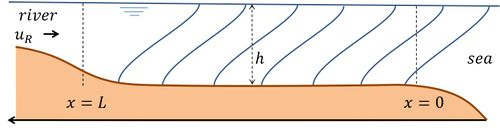Figure 1: Seawater intrusion in a longitudinal section of a prismatic estuary, represented by isohalines.

The classical theory of estuarine circulation was first developed by Hansen and Rattray in 1965 , after earlier investigations by D. Pritchard in the St. James river . Many other authors have subsequently contributed to further verification and refinement.

In the classical theory of estuarine circulation, advection of seawater up and down the estuary by the tidal motion and associated mixing processes are not considered explicitly. These models consider a stationary salinity field in a frame that moves cyclically up and down the estuary and a tide-averaged coefficient for the mixing processes. The effect of tidal motion is further restricted to turbulence generation at the bed, which enhances momentum dissipation and mixing in and across the bottom boundary layer.

## Notation

In the following, some basic notions of the classical theory are presented. We consider a straight prismatic estuary (uniform depth $h$ and width $b$ ); the width is small enough for neglecting the influence of earth's rotation (Rossby radius $U/fb \lt \lt 1$ ). We use the following notations (see also figure 1):

• $x$ is the coordinate along the estuarine axis ($x=0$ at the sea boundary, $x=L$ at the landward boundary of salt intrusion);
• $u$ is the velocity in the $x$-direction, $u(z) = u_R + u_C(z) + U \cos \omega t,$ where $u_R$ is the velocity related to river runoff (river discharge divided by estuarine cross-section), $u_C(z)$ is the estuarine circulation, $U$ is the depth-averaged tidal velocity amplitude and $\omega = 2 \pi / T$ is the tidal radial frequency;
• $S=s/s_0$ is the ratio of the local salinity $s$ and the seawater salinity $s_0 :$ $S$ is related to the density $\rho$ by the relation $\rho=\rho_0(1+\beta s_0 S),$ where $\rho_0$ is the fresh water density and the expansion coefficient $\beta=7.7 \times 10^{-4};$
• $p(z) = g \int_z^\eta \rho dz$ is the hydrostatic pressure; $\eta$ is the surface elevation relative to a horizontal plane and $-z$ is the depth relative to the surface;
• $K_M$ is the eddy viscosity (vertical momentum diffusion coefficient $[m^2/s]$ ) and $K_S$ the eddy diffusivity (vertical salinity diffusion coefficient).

Depth-averaging is denoted by an overbar.

## Assumptions

• The diffusion coefficients $K_M, K_S$ do not depend on the spatial and temporal coordinates $x,z,t$ ; this means that the classical model is realistic only for continuously stratified estuaries (no layered salinity structure; such estuaries are often called "partially mixed", see figure 1);
• The river flow velocity $u_R$ and the tidal velocity amplitude $U$ do not depend on the spatial coordinates $x,z$ ;
• The river flow velocity is much smaller than the tidal velocity, $u_R \lt \lt U$ ;
• The water surface variation is much smaller than depth, $\eta \lt \lt h$ ; Stokes drift is neglected;
• The vertical flow velocity $w$ is neglected;
• Side wall effects are neglected, implying uniformity of all variables in lateral $y$-direction;
• Longitudinal gradients in the velocity $u$ are neglected;
• The longitudinal salinity gradient is the same at all depths ($\large\frac{\partial S}{\partial x}\normalsize=\large\frac{\partial \overline S}{\partial x}\normalsize ).$

## Model formulation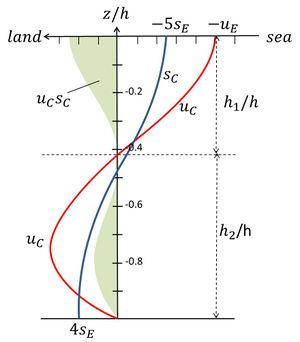Figure 2: Vertical profiles of estuarine circulation and salinity according to the classical theory (Eq. 4).

We consider the influence of a constant river flow $u_R$ on the salinity distribution in a frame moving with the velocity $u_R + U \cos \omega t$. In the moving frame the velocity $u$ is time-independent and depends only on the vertical coordinate $z$; we thus have $u=u_C(z)$. The only driving force acting on the fluid in the moving frame is the pressure gradient $\partial p / \partial x$, which is a linearly increasing function of depth ($-z$) below mean water level:

$\large\frac{1}{g \rho} \frac{\partial p}{\partial x}\normalsize = \large\frac{\partial \eta}{\partial x}\normalsize + (\eta -z) \beta s_0 \large\frac{\partial S}{\partial x}\normalsize . \qquad (1)$

The fluid acceleration caused by this pressure gradient is balanced by momentum dissipation through turbulent diffusion:

$\large\frac{\partial p}{\rho \partial x}\normalsize = \large\frac{\partial }{\partial z}\normalsize ( K_M \large\frac{\partial u_C}{\partial z}\normalsize) . \qquad (2)$

This equation can be solved considering the boundary conditions:

No slip at the bottom: $u_C(-h)=0$ , no momentum diffusion through the surface: $du_C / dz =0$ at $z=\eta \approx 0$ and no net discharge: $\int_{-h}^0 u_C dz =0$.

Because the river runoff is constant and the tide cyclic, the salinity distribution should not change with time in the moving frame. However, the estuarine circulation modifies the salinity distribution, because salt advection $u_C(z) S(x,z)$ has a gradient in $x$-direction. The salt balance is restored by vertical diffusion of salinity:

$u_C \large\frac{\partial \overline S}{\partial x}\normalsize = \large\frac{\partial }{\partial z}\normalsize ( K_S \large\frac{\partial S}{\partial z}\normalsize) . \qquad (3)$

This equation can be solved with the boundary conditions: no salt flux through bottom and surface, $\partial S / \partial z =0$ at $z=-h, z=0 .$

The solution of equations Eqs. (1-3) is given by (shown in figure 2):

$u_C(z)=u_E (1-9 \zeta^2-8 \zeta^3) , \; S=\overline S + s_C(z), \; s_C(z)=S_E (-5+30 \zeta^2-45 \zeta^4-24 \zeta^5), \; \zeta=z/h , \qquad (4)$

$u_E=\large\frac{g \beta s_0 h}{48 K_M} \frac{\partial \overline S}{\partial x}\normalsize , \; S_E=\large\frac{h^2 u_E}{60 K_S} \frac{\partial \overline S}{\partial x}\normalsize . \qquad (5)$

A frequently used estimate of the eddy viscosity in unstratified water is $K_M=hU_*^2/U,$ where $U_*=\sqrt{c_D}U$ is the friction velocity. We then find that the estuarine circulation $u_E$ is related to the Simpson stratification number $Si$ :

$u_E=\large\frac{U}{48}\normalsize Si , \quad Si=\large\frac{g}{\rho} \frac{\partial \rho}{\partial x} \frac{h^2}{U_*^2}\normalsize . \qquad (6)$

## Salt balance

The motion in the moving frame is not exactly cyclic; after one tide the moving frame has moved seaward over a distance $T u_R$ . In order to prevent complete flushing of the salinity a net landward salt transport should exist. Many seawater intrusion mechanisms can contribute to landward salt transport, as discussed in the article Seawater intrusion and mixing in estuaries. In this case, we assume that estuarine circulation is the primary mechanism for seawater intrusion. This means that the loss of salt content from the estuary due to the net shift of the moving frame, $Thu_rS$ , should be compensated by salt transport related to estuarine circulation:

$hu_RS=\int_{-h}^0 u_C(z)S(z) dz . \qquad (7)$

Evaluation of the integral gives, using Eqs. (4, 5),

$S=L^3 (\large\frac{\partial \overline S}{\partial x})^3\normalsize , \; L=0.024 h^2 c (\large\frac{c}{u_R K_S K_M^2})^{1/3}\normalsize , \; c=\sqrt{\beta s_0 gh} . \qquad (8)$

$L$ is an estimate for the length of the seawater intrusion due to estuarine circulation; $c$ is the internal wave speed in a fresh water layer of depth $h$ overlying seawater. By approximating $\partial \overline S / \partial x \approx -1 / L$ the maximum estuarine circulation velocity can be written as

$u_E=0.87(u_R c^2 \large\frac{K_S}{K_M})^{1/3}\normalsize. \qquad (9)$

In general $u_E\gt \gt u_R .$

## Stratification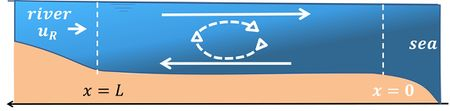Figure 3: Schematic representation of estuarine circulation in a prismatic channel.

According to equation (4), estuarine circulation corresponds to a net landward flow in a near bottom layer of thickness $h_2 \approx 0.58 h$ and a net seaward flow of the same order in the upper layer (see figure 3). By calling $s_1,Q_1$ the average salinity and average discharge in the upper layer and $s_2,Q_2$ the average salinity and discharge in the bottom layer, we can determine the average salinity difference $\Delta s = s_2-s_1$ ("stratification") from the condition of net zero salt transport:

$s_1Q_1 + s_2Q_2 =0 , \qquad (10)$

$Q_1 \approx -Q_2 - hbu_R \approx b \int_{-h_2}^0 u_C dz \approx -0.26hu_E . \qquad (11)$

This gives $\Delta s / s \approx 4.4 u_R / u_E . \qquad (12)$

The "bulk" Richardson number $Ri= \Delta \rho gh / U^2$ is a measure for the degree of stratification in an estuary. For small Richardson numbers (typically smaller than 0.25) the diffusion coefficients are not strongly influenced by vertical density gradients. In this case one may approximate $K_M \approx K_S \approx K = c_D hU.$ The friction coefficient $c_D$ is of the order of 0.002. Substitution in the expressions for $L$ (Eq. 8), $u_E$ (Eq. 9) and $\Delta s$ (Eq. 12) yields

$u_E=0.87 (u_R c^2)^{1/3} , \; \large\frac{\Delta s}{s}\normalsize = 4.4 (\large\frac{u_R}{c})^{2/3}\normalsize , \; L = \large\frac{0.024}{c_D}\normalsize (\large\frac{c}{u_R})^{1/3} \frac{hc}{U}\normalsize . \qquad (13)$

The estuarine circulation and the stratification increase with increasing river runoff; they do not depend on the tidal velocity.

In case of strong (but continuous) stratification the Richardson number is larger than 1. In this case the diffusion coefficients $K_M, K_S$ depend on $Ri$ and are strongly reduced following relations experimentally established by Munk-Anderson 

$K_M = K(1+10Ri)^{-1/2} \approx 0.316 K Ri^{-1/2} , \; K_S = K (1+3.3Ri)^{-3/2} \approx 0.167 K Ri^{-3/2} , \; Ri=(\large\frac{c}{U})^2 \frac{\Delta s}{s}\normalsize . \qquad (14)$

Substitution of these approximations yields

$u_E=0.3 U , \; \large\frac{\Delta s}{s}\normalsize = 13 \large\frac{u_R}{U}\normalsize , \; L = \large\frac{0.8}{c_D}\normalsize h (\large\frac{c}{U})^3 (\frac{u_R}{U})^{1/2}\normalsize . \qquad (15)$

With an increase of runoff $u_R$ the estuarine circulation $u_E$ remains unchanged, whereas the intrusion length $L$ increases. This counterintuitive result is due to the strong increase of stratification with increasing runoff.

## Observations

The assumption that seawater intrusion is due only to gravitational circulation strongly limits the salt intrusion length $L.$ The previous formulas indicate for realistic values $U=1 m/s, h=10 m, u_R=0.05 m/s, c_D=0.001-0.003$ an intrusion length $L \approx 0.5-6 km$. Observed salt intrusion lengths are typically several times larger, because other processes also contribute to seawater intrusion (see Seawater intrusion and mixing in estuaries). However, a much larger salt intrusion length (i.e. much smaller longitudinal density gradient) also implies a much smaller estuarine circulation $u_E$, according to equation (5). This is in contradiction with field observations, that show strong residual circulation (order 10 cm/s), even in the case of small longitudinal and vertical salinity gradients. Reasons for this discrepancy will be discussed is the following sections.

## Asymmetric Tidal Mixing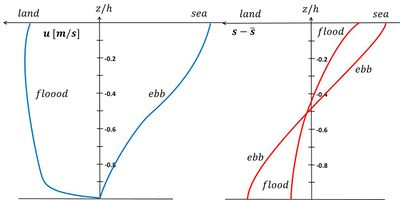Figure 4: Schematic representation of vertical profiles of salinity (right) and along-channel velocity (left) for flood and ebb.

In 1986, Van Aken  explained the onset of seasonal stratification in the North Sea by the process of tidal advection of surface water with lower density, related to the presence of strong salinity gradients. A few years later, Simpson et al.  observed and modeled tidal variation of stratification in Liverpool Bay by this differential advection mechanism, which they called "tidal straining". Numerical model studies by Jay and Musiak  and Scott  revealed that tidal straining can strongly influence estuarine circulation. When during flood high salinity seawater enters the estuary, the less dense estuarine surface water is repulsed. Vertical salinity stratification is therefore reduced, a process further strengthened in some cases by convective overturning (seawater being advected by tidal flow on top of less dense estuarine water ). For sufficiently weak stratification, the surface flow exchanges momentum with the near-bottom flow, which is accelerated in upstream direction. During ebb, the tidal flow restores stratification, by advecting less dense estuarine waters seaward over the denser near-bottom waters. The momentum exchange between surface and near-bottom water is now reduced; seaward tidal flow is concentrated near the surface, see figure 4. The net effect is an enhancement of residual landward near-bottom flow, as shown by numerical experiments .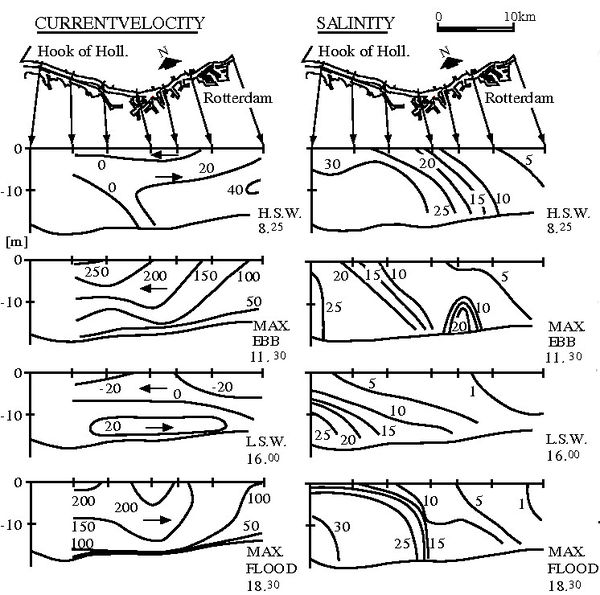Figure 5: Velocity and salinity distributions in a longitudinal section of Rotterdam Waterway on 9 September 1967 at different tidal phases: at the end of flood tide (H.S.W.=high slack water), during maximum ebb flow, at the end of ebb tide (L.W.S.-low slack water) and during maximum flood flow. The local salinity maximum halfway the estuary at maximum ebb is related to outflow of the Old Meuse tributary. Characteristics of Rotterdam Waterway are: approximately prismatic channel, width 300 m, depth 15 m, tidal range 2 m, maximum depth-averaged tidal velocity 1 m/s.

Enhancement of estuarine circulation by asymmetric tidal mixing only occurs in situations of weak stratification. In case of strong stratification (weak tides, high river runoff) momentum exchange between surface and near-bottom water hardly occurs (see Salt wedge estuaries). The major effect of weak tides in the latter case is an increase of eddy viscosity in the bottom layer, which reduces gravitational circulation . Enhancement of estuarine circulation by asymmetric tidal mixing is strongest for small Simpson numbers (typically $Ri \lt 1$) and periodic or weak stratification.

Figure 5 illustrates asymmetric tidal mixing in Rotterdam Waterway. The velocity and salinity profiles were measured on 9 September 1967 at an estimated net discharge of about 1000 m3/s (runoff velocity $u_R \approx 0.2 m/s$). Characteristic values of the Richardson and Simpson numbers are $Ri \approx 1.8, Si \approx 0.5 .$ The sections at maximum ebb and maximum flood flow display asymmetry between near-bottom flow velocities; near-bottom velocities are substantially higher during flood than during ebb (as schematically depicted in figure 4). The sections at H.S.W. and L.S.W. show asymmetry in stratification: at the end of the flood the water column is less stratified than at the end of the ebb. Another survey in Rotterdam Waterway was conducted by De Nijs et al. in 2006 at higher river discharge (stronger stratification) . These authors show that in Rotterdam Waterway asymmetry in tidal mixing is primarily related to asymmetry of the tide at the mouth (faster tidal rise than tidal fall, which enhances flood currents over ebb currents), more than to differential advection. This is consistent with the fast thickening of the bottom boundary layer during flood, caused by strong bed-generated turbulence. A similar behaviour was observed in the Hudson estuary .

## Lateral estuarine circulation

In natural estuaries the depth decreases towards the lateral boundaries (in a meander the depth decreases towards the inner channel bend). Tidal velocities are lower near the channel banks than at the channel centre. The salinity front is therefore not advected at the same speed at different lateral locations. This results in lateral salinity gradients. During flood (especially shortly before the end of the flood phase) the salinity is higher at the channel centre than near the channel banks. During ebb the situation is reversed; near the surface, the salinity at the channel centre is lower than near the channel banks. The lateral salinity gradients related to this differential tidal salinity advection, induce a baroclinic (density-driven) lateral current. This current has a vertical structure; during flood it is directed near the bottom toward the channel banks and toward the channel centre near the surface, see figure 6. The resulting flow convergence at the channel centre is often visible in the field by a scum line following the channel axis . During ebb the lateral exchange flow is divergent at the surface and convergent near the bottom. This lateral baroclinic exchange flow has important consequences for longitudinal dispersion in natural estuaries, as first pointed out by R. Smith , as explained in the article Seawater intrusion and mixing in estuaries.Figure 6: Lateral density-driven exchange flow related to cross-channel depth variation. Panel a: Cross-sectional salinity distribution and flow pattern shortly before the end of the flood phase. Higher salinity is indicated by darker blue. Panel b: The same in the last phase of ebb. The strength of along-channel flood flow in different parts of the cross-section is indicated by crossed circles; the strength of ebb flow by dotted circles. The lateral exchange flow influences the strength and the distribution of longitudinal estuarine circulation by altering the vertical distribution of along-channel flood and ebb flow. Panel c: Residual flow pattern (estuarine circulation). The crossed circle indicates up-estuary residual flow and the dotted circles down-estuary residual flow.

Lateral exchange flows also influence estuarine circulation. Downwelling flow at the channel axis during flood transports momentum down the vertical; surface flow is decelerated and near bottom flow accelerated in up-estuary direction. During ebb, vertical momentum transport reduces turbulent momentum dissipation by reducing the vertical shear of the tidal current. This also favours the baroclinic flow component . Lateral baroclinic exchange flow therefore enhances estuarine circulation. It also induces an a net downstream flow component in the shallower parts of the cross-section (lateral inflow of down-estuary momentum from the channel centre during ebb, outflow of up-estuary momentum toward the channel centre during flood, see figure 6); this flow component enhances the down-estuary flow component related to river runoff .

## Other lateral circulation patterns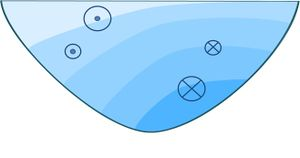Figure 7: Influence of earth's rotation on estuarine circulation. The up-estuary residual flow (crossed circles) is shifted toward the right channel bank (looking in up-estuary direction, northern hemisphere) and the down-estuary residual flow (dotted circles) toward the left bank.

Several mechanisms can alter the symmetric flow pattern of figure 6. In wide estuaries the symmetry is broken by earth's rotation. Currents are deviated to the right on the northern hemisphere and to the left on the southern hemisphere (see Coriolis acceleration). Looking in up-estuary direction, flood currents are concentrated at the right side of a straight tidal channel and ebb currents at the left side (northern hemisphere). Estuarine circulation is influenced by earth's rotation in a similar way : the residual up-estuary flow is shifted to the right bank and the down-estuary flow to the left bank, see figure 7. The mean cross-sectional salinity distribution is tilted accordingly.

Channel meandering induces transverse circulation, directed to the inner channel bend in the lower part of the vertical and to the outer bend near the surface. The spiraling tidal flow modifies the salinity distribution and the density-driven longitudinal residual flow in a similar way as earth's rotation when the tidal flow follows a channel meander with the inner channel bend at the right bank . For an inner channel bend at the left bank the picture is mirrored; in this case the effects of channel meandering and earth's rotation are opposite. Channel bends and other topographic structures (bars, shoals) generate longitudinal circulating flow patterns in estuaries also in the absence of density differences. These horizontal topographically induced flow circulations are often stronger than horizontal estuarine circulation generated by density gradients. However, residual flow circulation in the longitudinal-vertical plane is mainly due to the density difference between seawater and river water.

## Consequence of estuarine circulation

Estuarine circulation is highly relevant for transport of fine sediments and sedimentation (see Dynamics of mud transport). The up-estuary near-bottom flow captures a substantial part of the downstream flux of fluvial sediment, and moves this sediment back toward the tip of the salt intrusion zone. The up-estuary near-bottom flow contributes also to import of marine sediment into the estuary. This sediment settles on the bed during periods of neap tide and slack water. It is resuspended in periods of high tidal currents, leading to a strong increase in turbidity. Decomposition of organic material in the high turbidity zone can cause oxygen depletion, especially in the lower part of the vertical where mixing with oxygen-rich surface water is inhibited by stratification. However, estuarine circulation is not the only cause of up-estuary sediment transport. Many field and model investigations show that tidal asymmetry due to non-linear tidal propagation in shallow water (leading to stronger flood currents than ebb currents and longer high-water slack periods than low-water slack periods) is often a more important mechanism, see the article Tidal asymmetry and tidal basin morphodynamics.

## Related articles

Salt wedge estuaries
Seawater intrusion and mixing in estuaries
Coriolis acceleration
Dynamics of mud transport